Test: Lines, Angles & Triangles - 1

# Test: Lines, Angles & Triangles - 1

Test Description

## 10 Questions MCQ Test Mathematics for Class 5 | Test: Lines, Angles & Triangles - 1

Test: Lines, Angles & Triangles - 1 for Class 5 2023 is part of Mathematics for Class 5 preparation. The Test: Lines, Angles & Triangles - 1 questions and answers have been prepared according to the Class 5 exam syllabus.The Test: Lines, Angles & Triangles - 1 MCQs are made for Class 5 2023 Exam. Find important definitions, questions, notes, meanings, examples, exercises, MCQs and online tests for Test: Lines, Angles & Triangles - 1 below.
Solutions of Test: Lines, Angles & Triangles - 1 questions in English are available as part of our Mathematics for Class 5 for Class 5 & Test: Lines, Angles & Triangles - 1 solutions in Hindi for Mathematics for Class 5 course. Download more important topics, notes, lectures and mock test series for Class 5 Exam by signing up for free. Attempt Test: Lines, Angles & Triangles - 1 | 10 questions in 10 minutes | Mock test for Class 5 preparation | Free important questions MCQ to study Mathematics for Class 5 for Class 5 Exam | Download free PDF with solutions
 1 Crore+ students have signed up on EduRev. Have you?
Test: Lines, Angles & Triangles - 1 - Question 1

### How many of the following figures have parallel lines?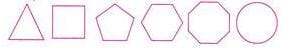Detailed Solution for Test: Lines, Angles & Triangles - 1 - Question 1

As shown in above figures, figure 2, figure 4 and figure 5 are the figures which has  the pair of parallel lines

Hence the correct answer is option B

Test: Lines, Angles & Triangles - 1 - Question 2

### How many pairs of parallel lines are there in the figure?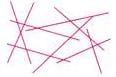Detailed Solution for Test: Lines, Angles & Triangles - 1 - Question 2

Pair of parallel lines in above figure  2 pair of parallel lines.

Test: Lines, Angles & Triangles - 1 - Question 3

### Four pupils each made a statement about the given figure.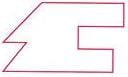Jyoti : "I can see 6 pairs of perpendicular lines". Harsha: "There are 7 angles in the figure". Jiya : "There is only one pair of parallel lines". Harsh : "6 of the angles are less than a right angle". Which pupil make the correct statement?

Detailed Solution for Test: Lines, Angles & Triangles - 1 - Question 3

Here, 6 pairs of perpendicular lines are CB⊥ AB, BC ⊥ CD, ED ⊥ CD, DE⊥ EF, FG⊥ EF and FG ⊥ HG.

Hence Jyoti made the correct statement..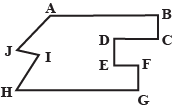Test: Lines, Angles & Triangles - 1 - Question 4

How many of the given letters have perpendicular lines?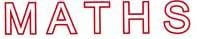Detailed Solution for Test: Lines, Angles & Triangles - 1 - Question 4

Letters M, T and H have perpendicular lines.

Test: Lines, Angles & Triangles - 1 - Question 5

The clock shows the time in the morning. ∠X represents ____ angle.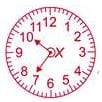Detailed Solution for Test: Lines, Angles & Triangles - 1 - Question 5

Any angle which is larger than 180o but smaller than 360o is called as a reflex angle.

Here, the angle shown is larger than 180o but smaller than 360o.

Hence, it is a reflex angle.

Therefore, option C is correct.

Test: Lines, Angles & Triangles - 1 - Question 6

At 3:25, the smaller angle formed between the two hands of a clock is ____.

Detailed Solution for Test: Lines, Angles & Triangles - 1 - Question 6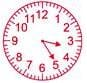Test: Lines, Angles & Triangles - 1 - Question 7

Which type of angle best describes angle Q?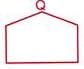Detailed Solution for Test: Lines, Angles & Triangles - 1 - Question 7

Measure of angle Q is more thaan 90o but less than 180o.

Hence the ∠Q is an obtuse angle.

Test: Lines, Angles & Triangles - 1 - Question 8

There are six angles below. How many of them are greater than a right angle?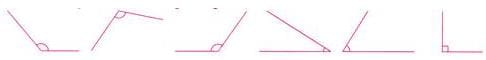Detailed Solution for Test: Lines, Angles & Triangles - 1 - Question 8

Figure A, Figure B and Figure C are the angles that are greater than the right angle.

Hence the correct answer is option C

Test: Lines, Angles & Triangles - 1 - Question 9

Which of the following is greater than a right angle?

Detailed Solution for Test: Lines, Angles & Triangles - 1 - Question 9

The question asks us to mark the correct option in which the angle formed by the two lines is greater than a right angle which means greater than 90∘90∘. To solve the question we need to move anticlockwise from the horizontal line to the other line and check whether the angle is greater than 90∘90∘ or not.
So on analysing option A we see that the angle formed between the two lines is 90∘90∘, as the lines are perpendicular. The image shows that the two lines are horizontal and vertical.
On analysing the second option we see that when we move anticlockwise along the line we see that the angle formed is less than 90∘90∘.
Moving to option C, since the angle is measured anticlockwise so on measuring the angle we see that the angle is present in the 3rd3rd quadrant. The angle formed is less than 90∘90∘as we move anticlockwise along the horizontal line given in the image.
In the fourth option we see that the angle formed on moving your hand anti-clockwise along the horizontal line we get an angle which is greater than 90∘90∘also called obtuse angle.

So, the correct answer is “Option D”.

Test: Lines, Angles & Triangles - 1 - Question 10

An angle which measures more than 90 but less than 180∘ is called_____.

Detailed Solution for Test: Lines, Angles & Triangles - 1 - Question 10

An obtuse angle is an angle whose measure is greater than 90 degrees but less than 180 degrees.

## Mathematics for Class 5

4 videos|55 docs|35 tests
Information about Test: Lines, Angles & Triangles - 1 Page
In this test you can find the Exam questions for Test: Lines, Angles & Triangles - 1 solved & explained in the simplest way possible. Besides giving Questions and answers for Test: Lines, Angles & Triangles - 1, EduRev gives you an ample number of Online tests for practice

## Mathematics for Class 5

4 videos|55 docs|35 tests(Scan QR code)